April 23, 2019

Neural Networks from scratch in python

I recommend you to read the tutorials about Logistic regression tutorial and decision trees tutorial due to the fact I explain important topics in those tutorials that we will use here.

As we have seen, the logistic regression model is used to resolve classification problems, this model separates the classes with a single decision surface (the white line in the image below), in fact, logistic regression is a linear classifier model, for instance this model is useful if we are working with linearly separable classes like in the image below: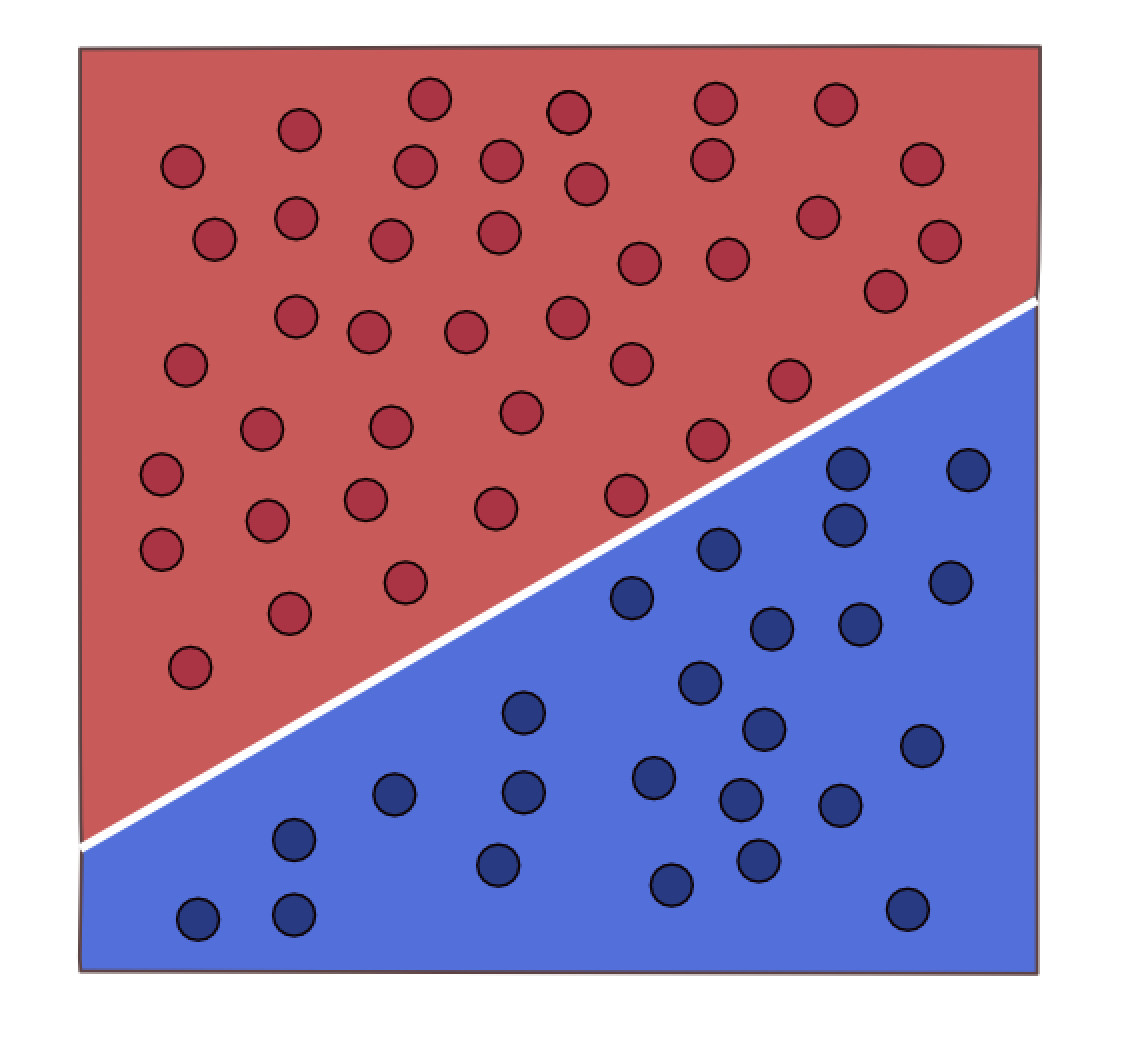Linearly separable classes

However, sometimes our classes are not linearly separable, like in the following image: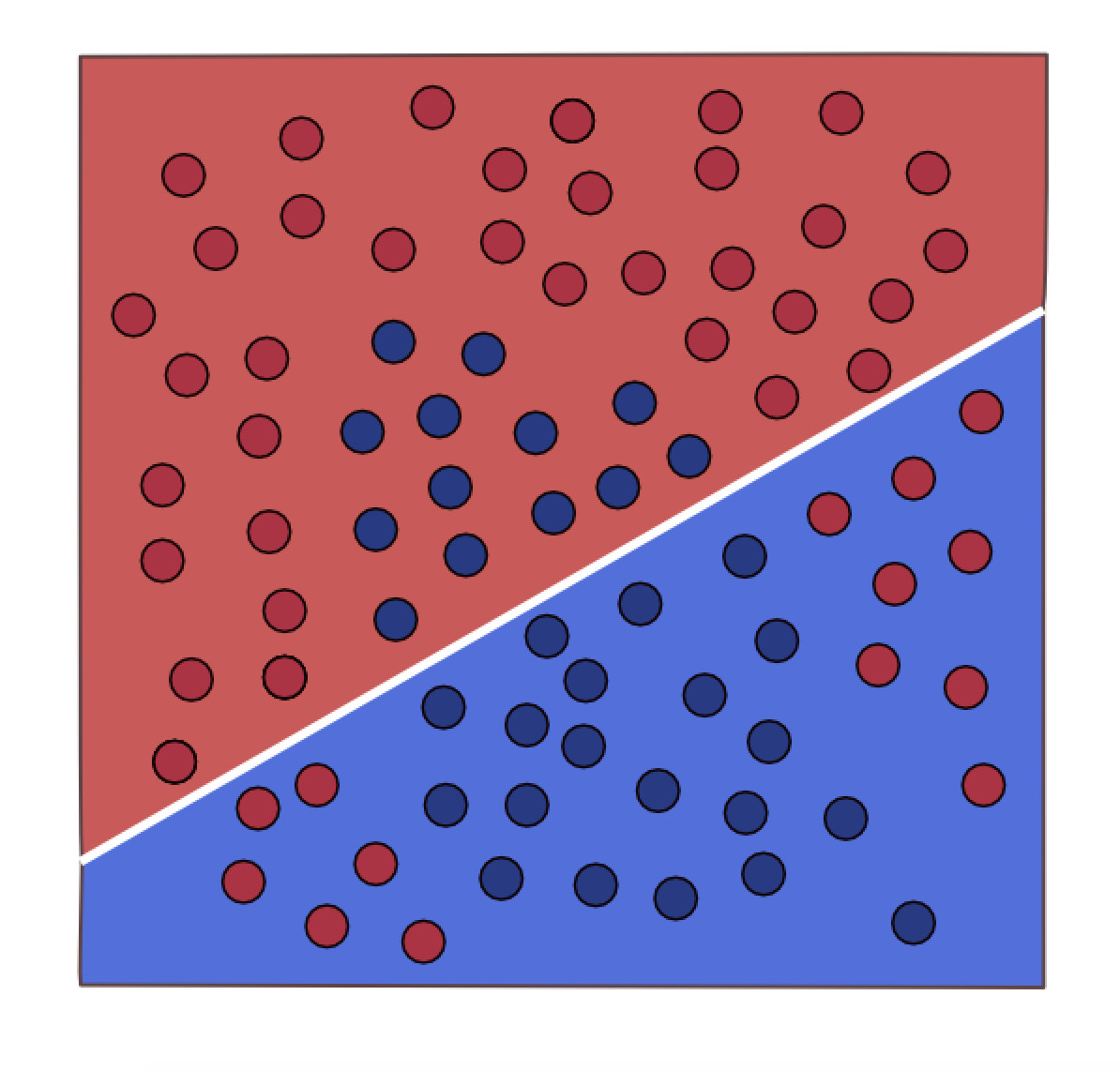Not linearly separable classes

We can not use a logistic regression model to classify this data. Neural networks aims to solve this problem.

In the following image we have a visual representation of the logistic regression model: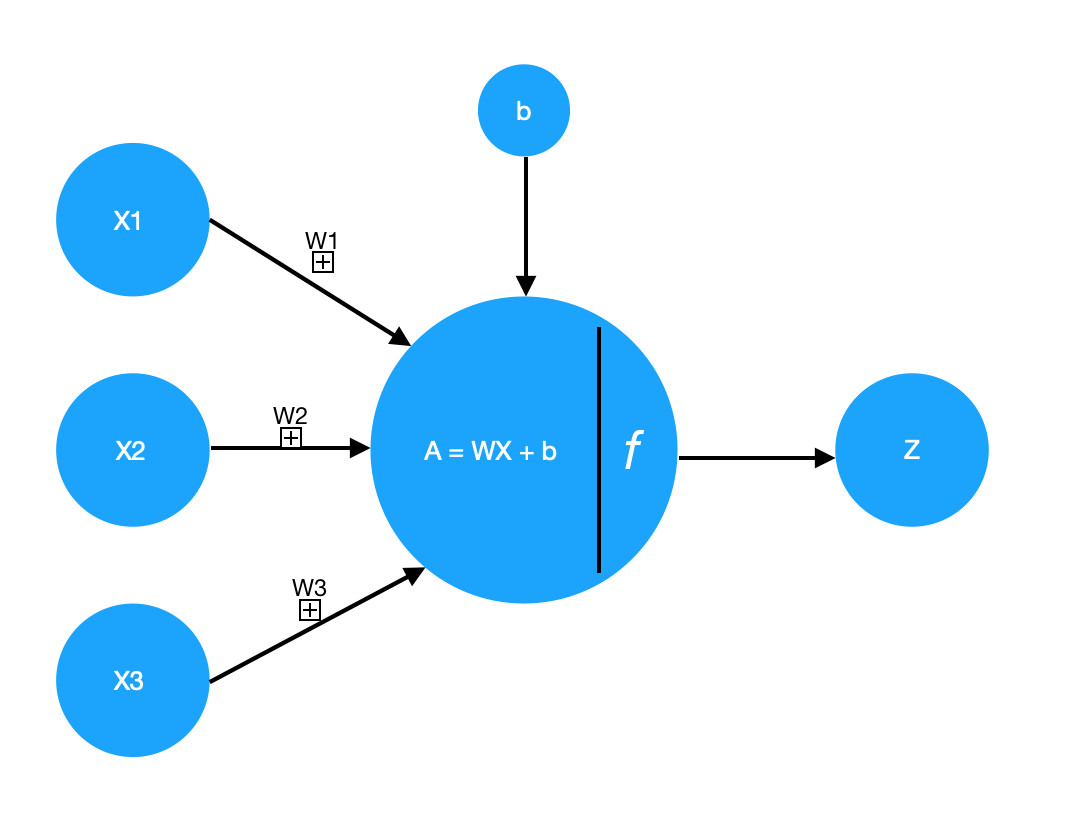Where Z is the result of the probability function and A is the result of applying the sigmoid function to Z, as we can remember A is the class predicted by the model.

We call this representation a neuron, a neural network, as the name suggest, has several neurons: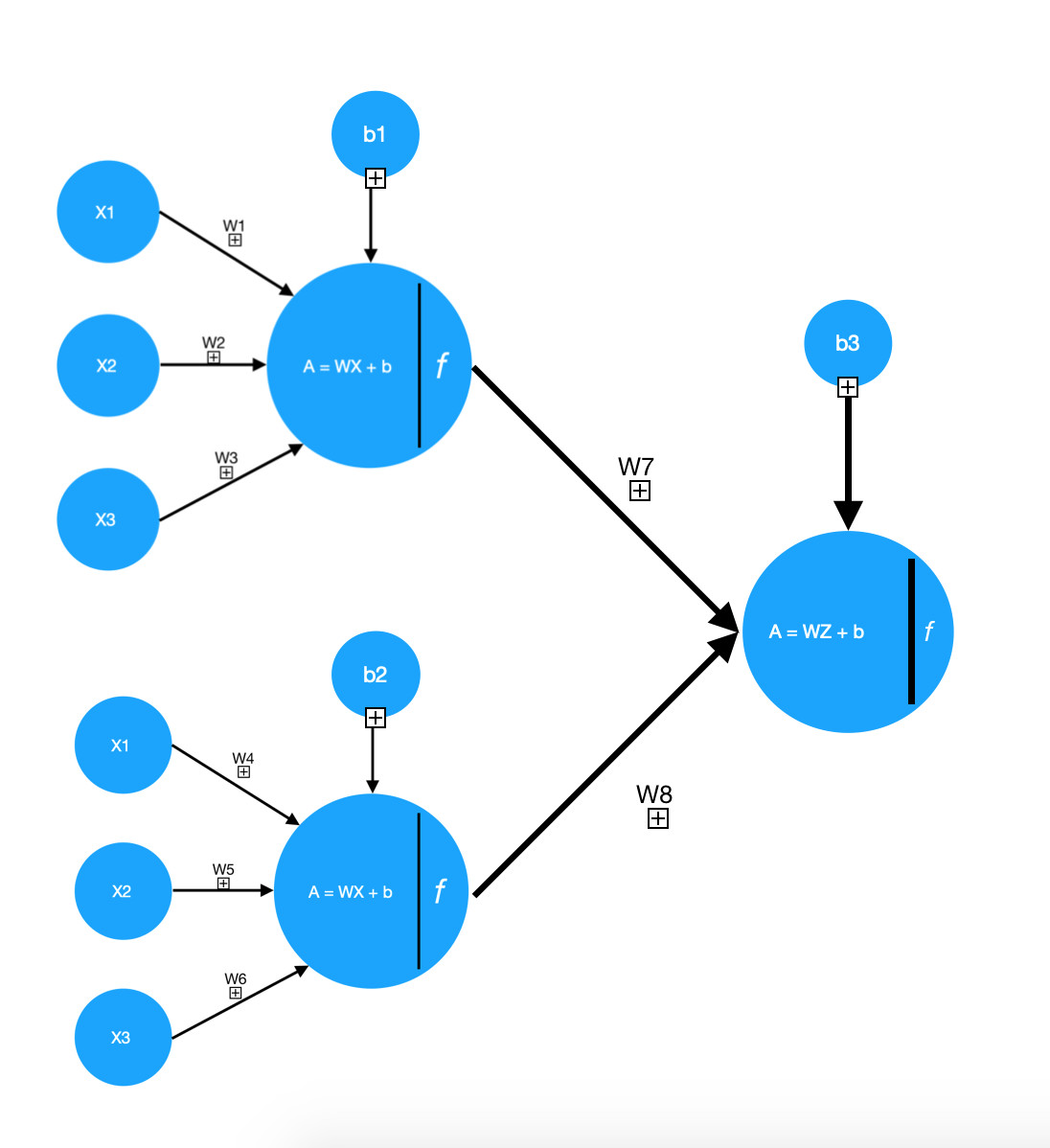Now we have two logistic regression models or two neurons and one output neuron, these two neurons will split the data in different ways:

First neuron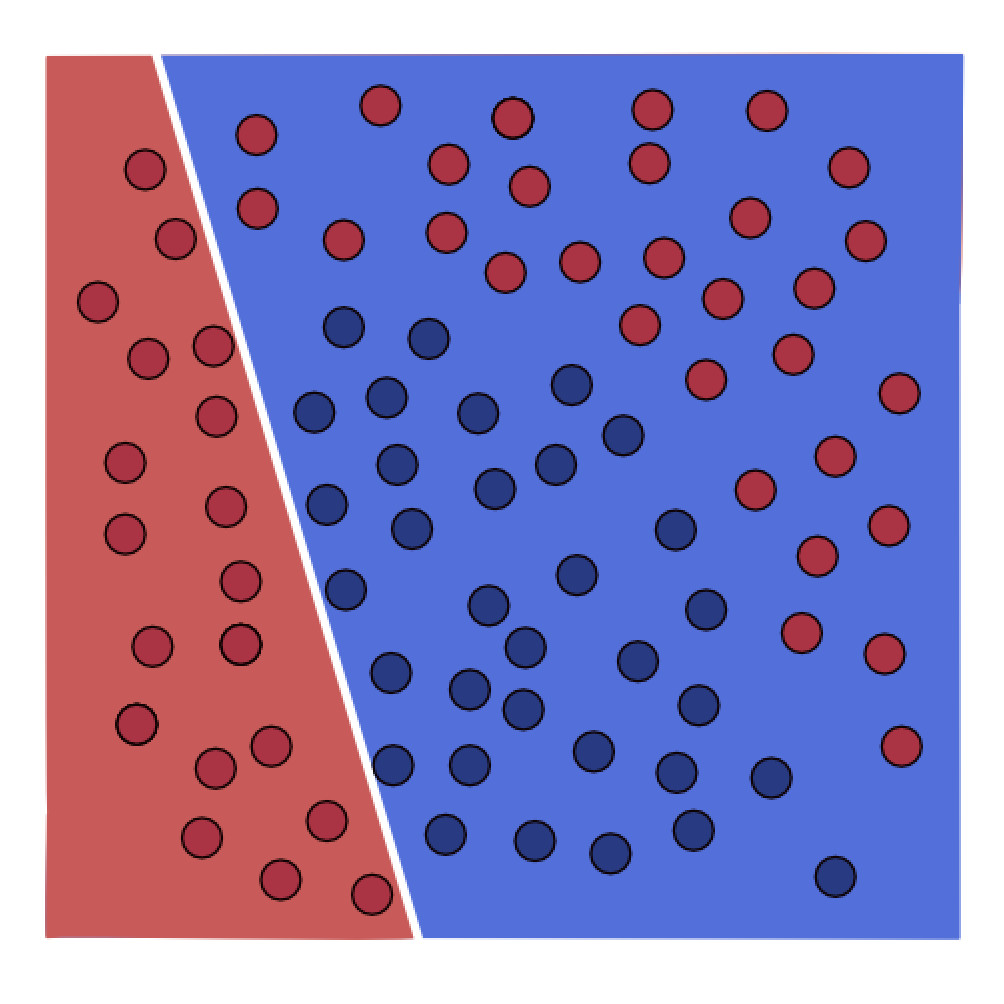Second neuron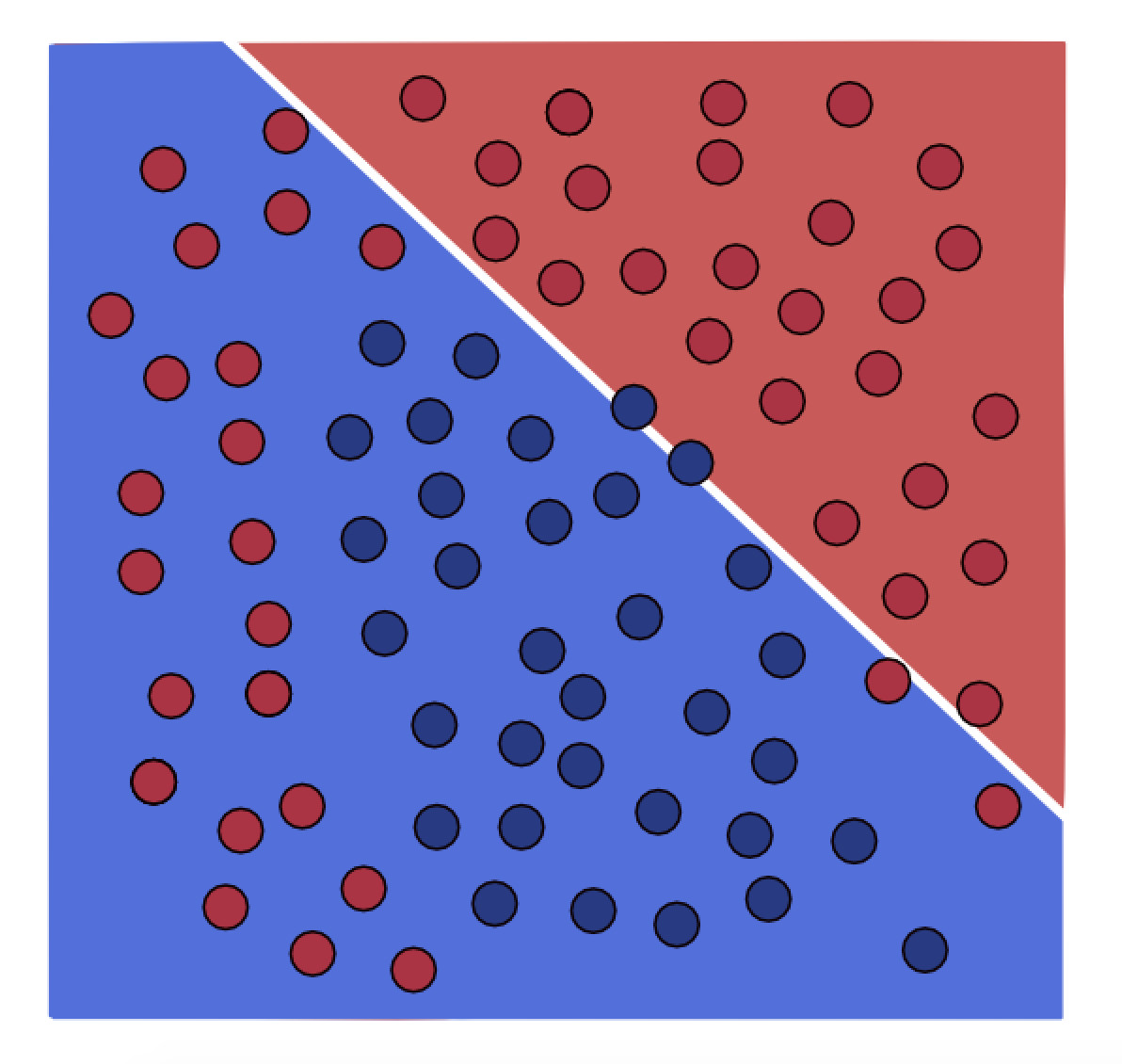The output neuron will merge these both neurons to create a more complex model: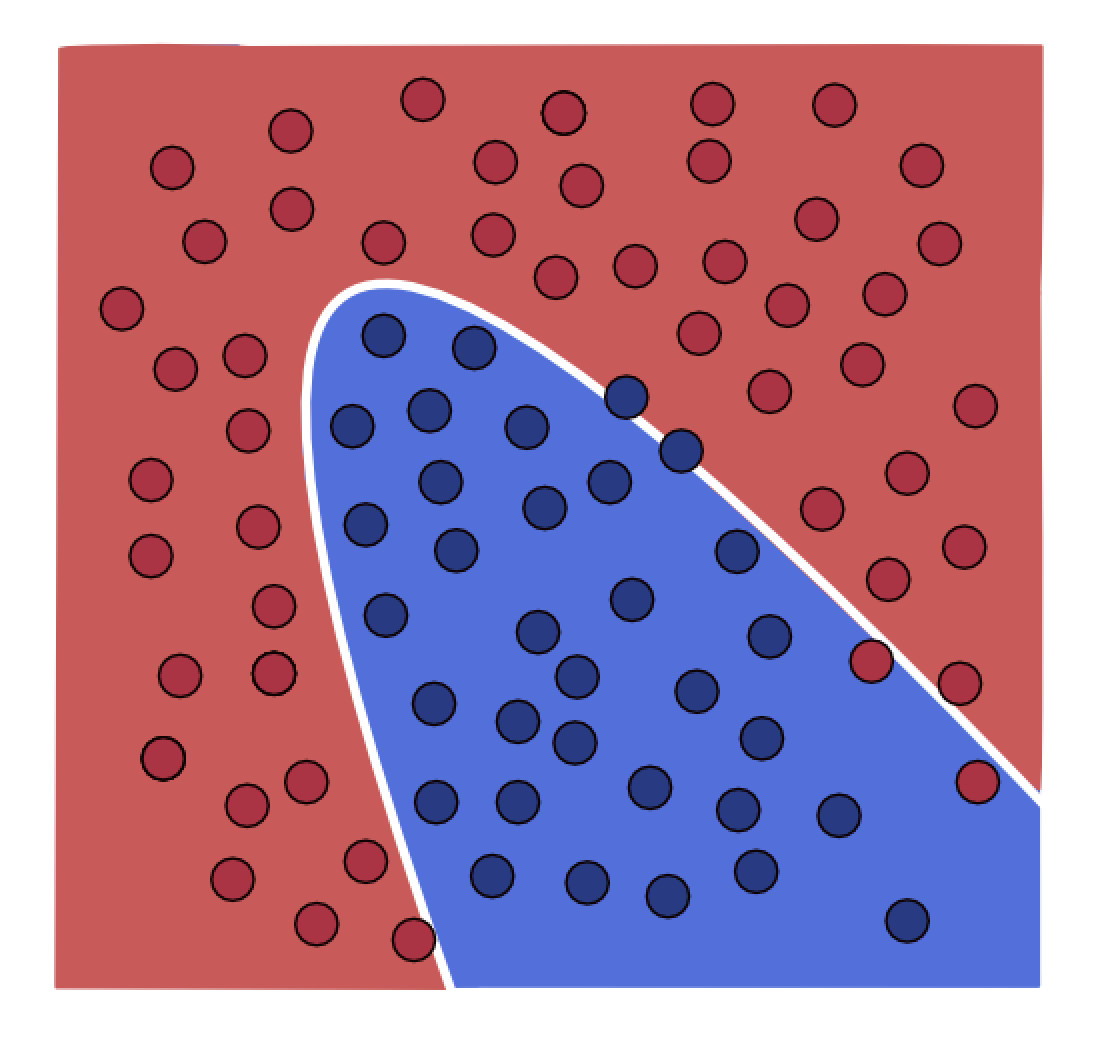Therefore we could say that a neural network is made of several logistic regression models. Usually we represent a neural network like in the image below: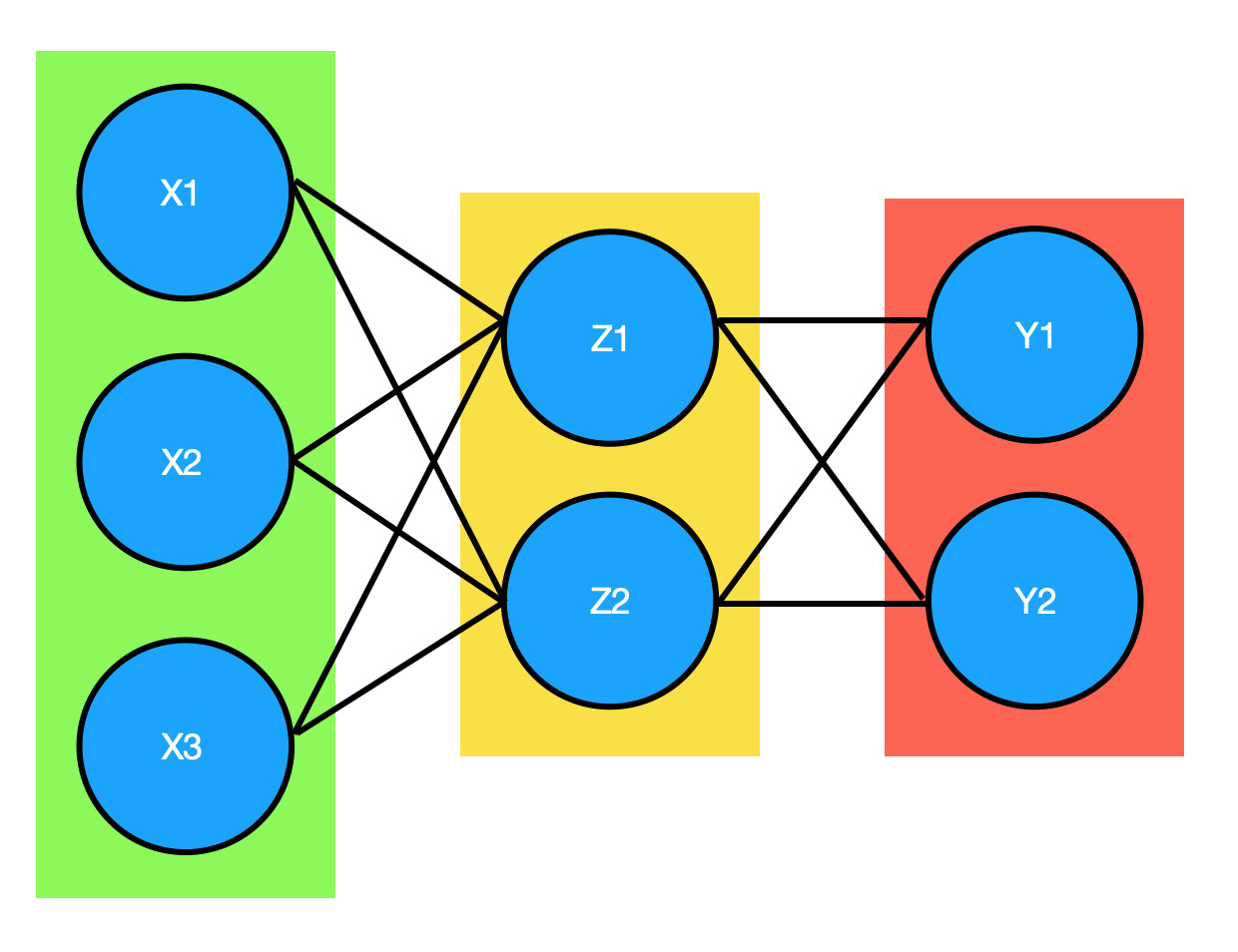In this representation each black line represents a variable W and the blue circles are neurons, we group these neurons in different clusters that we call layers:

• Green layer: We call this layer the input layer, this layer contains the input data X.
• Yellow layer: This layer is called the hidden layer, we can have several hidden layers in a neural network with different amount of neurons, if we have more of these layers the model will be more complex.
• Red layer: This layer is called the output layer, the output of this layer is the score of each class if we are resolving a classification problem. The number of nodes in this layer represents the number of classes we have in the dataset, although we can use only one neuron if we have two classes.

Each layer takes as input the output of the previous layer and as I mentioned we can have more complex neural networks like the following one: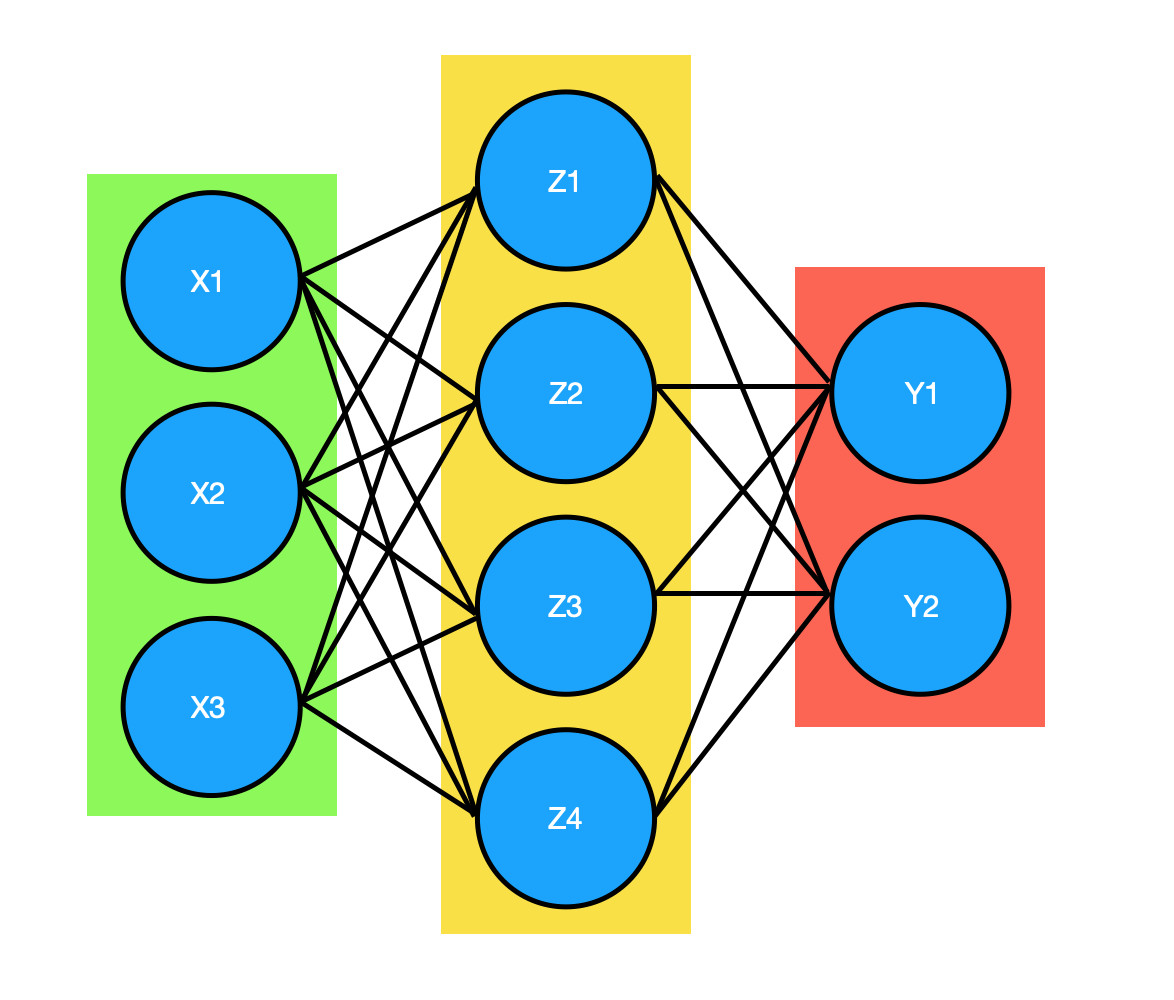This neural network has more neurons in the hidden layer.

Python code

Now we are going to create a neural network from scratch, you can see the complete code in this notebook

Libraries

import matplotlib.pyplot as plt
import numpy as np

from sklearn import datasets
from sklearn.model_selection import train_test_split

The dataset

X, y = datasets.make_moons(300, noise=0.20)
plt.scatter(X[:,0], X[:,1], s=40, c=y, cmap=plt.cm.Spectral)

We created a non linear dataset like in the example below: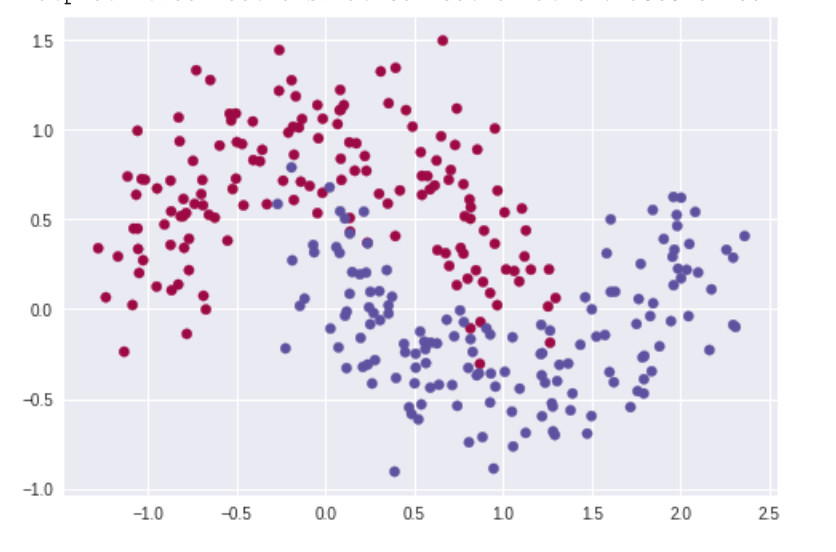We can say that each point is a register in the dataset, it could be a person, an image, etc.

Functions

def sigmoid(Z):
return 1 / ( 1 + np.exp(-Z))

This is the sigmoid function that we saw in the logistic regression tutorial, we will use it in the same way, to predict the class of each data point.

Activation function ReLU

def relu(Z):
return np.maximum(0, Z)

This function returns 0 if the value (Z) is less or equal to 0 and returns the value (Z) if (Z) is greater than 0.

We use this function as an activation function, in a neural network each node will learn a different patter, for example if we are classifying digits (0 to 9), some neurons will learn to identify the patterns that the digit 8 has, if the neural network takes as input an image of the digit 8 these neurons will have a value greater than 0 and the activation function will return this value, we can say that the activation function turned on these neuron, however if the neural network takes as input an image of the digit 7 these neurons will have a value smaller than or equal to 0 and the activation function will return 0 then we can say that the activation function turned off these neurons, finally the neural network knows what neurons predict if the input digit is 8 and if it sees these neurons turned on the neural network will know that the digits is 8.

def prime_relu(Z):
return np.heaviside(Z, 0)

This is the derivative of the relu function, we need it to compute the backpropagation step.

def forward_propagation(X, W1, b1, W2, b2):
forward_params = {}

Z1 = np.dot(W1, X.T) + b1
A1 = relu(Z1)
Z2 = np.dot(W2, A1) + b2
A2 = sigmoid(Z2)

forward_params = {
"Z1": Z1,
"A1": A1,
"Z2": Z2,
"A2": A2,
}

return forward_params

In the forward propagation step we compute the multiplications of the parameter W and the input data X as we did in the logistic regression model but this time we have two layers consequently we need to compute two multiplications.

We need to return the variables Z1, A1, Z2, A2 to compute the backpropagation step.

def loss_function(A2, y):
data_size = y.shape
cost = (-1 / data_size) * (np.dot(y, np.log(A2).T) + np.dot(1 - y, np.log(1 - A2).T))
return cost

Here we have the loss or cost function, this loss function is called binary cross entropy and we use it when we have two classes in the dataset. As I mentioned in the logistic regression tutorial, we use this function to measure the performance of the neural network.

def backward_propagation(forward_params, X, Y):
A2 = forward_params["A2"]
Z2 = forward_params["Z2"]
A1 = forward_params["A1"]
Z1 = forward_params["Z1"]

data_size = Y.shape

dZ2 = A2 - Y
dW2 = np.dot(dZ2, A1.T) / data_size

db2 = np.sum(dZ2, axis=1) / data_size

dZ1 = np.dot(dW2.T, dZ2) * prime_relu(Z1)
dW1 = np.dot(dZ1, X) / data_size
db1 = np.sum(dZ1, axis=1) / data_size

db1 = np.reshape(db1, (db1.shape, 1))

"dZ2": dZ2,
"dW2": dW2,
"db2": db2,
"dZ1": dZ1,
"dW1": dW1,
"db1": db1,
}

I have a post where I explain this part.In this case we are using the relu activation function and not the sigmoid function but the main idea is the same.

def one_hidden_layer_model(X, y, epochs=1000, learning_rate=0.003):
np.random.seed(0)
input_size = X_train.shape
output_size = 1
hidden_layer_nodes = 5

W1 = np.random.randn(hidden_layer_nodes, input_size) / np.sqrt(input_size)
b1 = np.zeros((hidden_layer_nodes, 1))
W2 = np.random.randn(output_size, hidden_layer_nodes) / np.sqrt(hidden_layer_nodes)
b2 = np.zeros((output_size, 1))

loss_history = []

for i in range(epochs):
forward_params = forward_propagation(X, W1, b1, W2, b2)
A2 = forward_params["A2"]
loss = loss_function(A2, y)

if i % 1000 == 0:
loss_history.append(loss)
print ("Costo e iteracion %i: %f" % (i, loss))

return W1, b1, W2, b2

Here we have the complete neural network code, I will explain each part:

input_size = X_train.shape
output_size = 1
hidden_layer_nodes = 5
• input_size: How many neurons the input layer has.
• output_size: How many neurons the output layer has.
• hidden_layer_nodes: How many neurons the hidden layer has.

The size of these variables has to match the size of the W and b variables:

W1 = np.random.randn(hidden_layer_nodes, input_size) / np.sqrt(input_size)
b1 = np.zeros((hidden_layer_nodes, 1))
W2 = np.random.randn(output_size, hidden_layer_nodes) / np.sqrt(hidden_layer_nodes)
b2 = np.zeros((output_size, 1))

In the code below we compute the forwardpropagation step and the backpropagation step.

for i in range(epochs):
forward_params = forward_propagation(X, W1, b1, W2, b2)
A2 = forward_params["A2"]
loss = loss_function(A2, y)

if i % 1000 == 0:
loss_history.append(loss)
print ("cost and epoch %i: %f" % (i, loss))

Training the neural network

In order to train a neural network, first we have to create the training and validation sets:

X_train, X_val, y_train, y_val = train_test_split(X, y, random_state=0, test_size=0.20)
y_train = np.reshape(y_train, (1, y_train.shape))
y_val = np.reshape(y_val, (1, y_val.shape))

We train the neural network with the code below:

W1, b1, W2, b2 = one_hidden_layer_model(X_train, y_train, epochs=20000, learning_rate=0.003)

Now we can use the W and b variables to make predictions:

def predict(W1, b1, W2, b2, X):
data_size = X.shape
forward_params = forward_propagation(X, W1, b1, W2, b2)

y_prediction = np.zeros((1, data_size))

A2 = forward_params["A2"]

for i in range(A2.shape):
y_prediction[0, i] = 1 if A2[0, i] > 0.5 else 0

return y_prediction
train_predictions = predict(W1, b1, W2, b2, X_train)
validation_predictions = predict(W1, b1, W2, b2, X_val)

print("train accuracy: {} %".format(100 - np.mean(np.abs(train_predictions - y_train)) * 100))
print("test accuracy: {} %".format(100 - np.mean(np.abs(validation_predictions - y_val)) * 100))
train accuracy: 85.41666666666667 %
test accuracy: 83.33333333333334 %

We can tune a lot of hyperparameters of this neural network, we can change the number of neurons, the number of hidden layers, the value of the learning rate, the activation function.

There are several frameworks to create neural networks like Keras or TensorFlow, we can create neural networks from scratch to see how they work, however it's a bad idea to use this neural networks to solve problems, we could have errors or make a mistake that will take us some time to fix, in the next tutorial we will use Keras to create a neural network.